• 倒相管和放大管
千次阅读
2020-12-14 22:00:54

## 晶体管与场效应管的区别

这是一点点个人读书时候摘抄的笔记，只希望能够帮到你。来源——《模拟电子技术基础》（第五版，董诗白，高等教育出版社）侵删。

场效应晶体管的栅极G、源极S、漏极D对应于晶体管的基极B、发射极E、集电极C，它们的作用相类似。

一、场效应管用栅-源电压U-GS控制漏极电流I-D，栅极基本不取电流。而晶体管工作的时候基极总是要索取一定的电流。因此，要求输入电阻高的电路应选用场效应管；而若信号源可以提供一定的电流，则可选用晶体管。

二、场效应管只有多子参与导电。晶体管内既有多子又有少子参与导电，而少子数目受温度、辐射等因素影响很大，因而场效应晶体管比晶体管的温度稳定性好、抗辐射能力强。所以在环境条件变化很大的情况下应选用场效应晶体管。

三、场效应晶体管的噪声系数很小，所以低噪声放大器的输入级要求信噪比较高的电路应该选用场效应晶体管。当然也可以选用特制的低噪声晶体管。

四、场效应管的漏极与源极可以互换使用，互换后特性变化不大。而晶体管的发射极与集电极互换后特性差异很大，因此只在特殊需要时才互换，成倒置状态，如在集成逻辑电路中。

五、场效应晶体管比晶体管的种类多，特别时耗尽型MOS管，栅-源电压U-GS可正、可负、可零，均能控制漏极电流。因而在组成电路时场效应晶体管比晶体管更加的灵活。

六、场效应晶体管和晶体管均可用于放大电路和开关电路，它们构成了品种繁多的集成电路。但由于场效应晶体管集成工艺更简单，且具有耗电低、工作电源电压范围宽等优点，因此场效应晶体管被越来越多的用于大规模和超大规模集成电路之中。

更多相关内容
• 在音频前置放大器中，并联调整推挽（ShuntRegulatedPush-Pull，SRPP）电路具有高增益、低失真、低输出阻抗等特点，能够获得优异的音质表现，因而在音响电路中广泛应用。本文设计了一款以共阴极放大器为输入级，SRPP...
•   差动放大电路又叫差分放大电路，它除了能放大交流信号，也能放大直流信号...  与之前学习的晶体管放大电路相比，差分放大电路形态上最大的特点是两个输入，两个输出。所谓差分，指的是电路放大的是两个输入的...

差动放大电路又叫差分放大电路，它除了能放大交流信号，也能放大直流信号，还能有效地减小由于电源波动和晶体管随温度变化而引起的零点漂移（温度漂移），因而获得广泛的应用。差分放大电路是架在晶体管与芯片之间的“桥梁”，比如在运算放大器的初级（输入端），一般来说会有差分放大电路。
与之前学习的晶体管放大电路相比，差分放大电路形态上最大的特点是两个输入，两个输出。所谓差分，指的是电路放大的是两个输入的电压差。之前的放大电路都是放大交流信号，差分放大电路可以放大电压差，即不但可以放大交流信号，也可以放大直流信号。我们本节将观察输入1KHz，100毫伏峰峰值）的正弦波时，在两个输出端各有什么现象。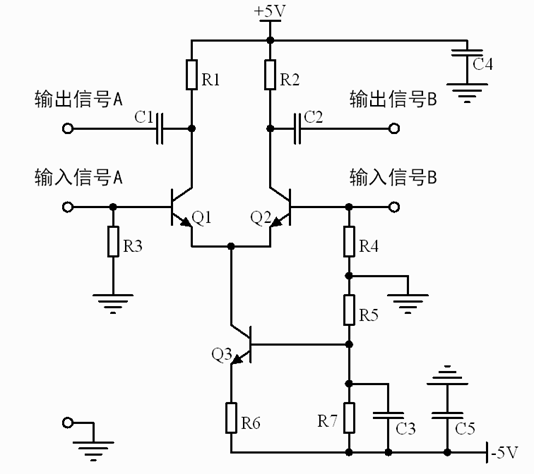差分放大电路是在共射极放大电路上改进得到的，它需要2个三极管像“镜子”一样工作：一样的型号，一样的外围电路，一样的工作特性（放大倍数，发射结电压，温度特性都相同）。

### 电流源与负电源

在电路图中，三极管Q3基极与发射极的电压V_BE3是不变的，偏置电阻R5与R7的值也不变，R5与R7经过分压得到的基极电位V_B3随之也能确定下来，R6的阻值也确定，那么Q3的基极与发射极之间的电流I_C3也是一定的——它只取决于Q3的工作状态，与输入的信号几乎没有任何关系。分析I_C3的方向，是“从上到下”，电流流入Q3的集电极，所以我们也称Q3将吸取电流，且电流大小不变，所以，Q3是作为恒流源来工作的。
所谓恒流源，顾名思义，是电流大小保持不变，而理想的恒流源应该具有以下特点：
1、输出电压不因负载变化而改变；
2、不因环境温度变化而改变；
3、内阻为无限大（以使其电流可以全部流出到外面）。
此处的Q3，作用就是保证吸取的集电极电流大小是不变的。这个结论是分析Q1与Q2工作的桥梁，非常重要。也是理解这个电路的关键
观察原理图还可以发现，电路中使用了-5V的电源。这是为了保证Q1与Q2的基极电压是0V，以实现直流的放大。此时，输入端也无需耦合电容了。如果不使用负电源，那么输入端需要耦合电容，就不能放大直流；同时Q1与Q2的基极偏置电路设计也会变得非常麻烦。所以一般使用负电源来进行设计。

### 两个共射放大电路

差分放大电路的工作秘密在于使用了Q3作为电流源，由于吸取的集电极电流大小是不变的，那么流过Q1与Q2的电流的和就确定了。
由于Q1与Q2的工作特性（理论上来讲）完全一致，所以，在没有任何输入信号的时候，各自的发射极电流也相等。如果设电流源的吸收的电流是2I_E的话：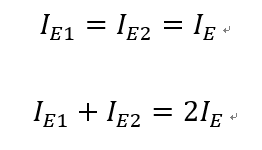如果在“输入信号A”加正电压，“输入信号B”输入0V，那么I_E1将会变大，假设I_E1的增加量为ΔI，那么I_E2的减小量也是ΔI，电流的变化将以压降的形式从电阻R1与R2上取出，如果电流的变化大小相同，方向相反，那么R1与R2上电压的变化量也一定大小相同，方向相反。
对于Q1组成的放大电路（这是一个共射极放大电路），“输出信号A”与“输入信号A”相比，波形被反相放大；对于Q2组成的放大电路，虽然此时“输入信号B”没有信号，但是“输入信号A”带来的电流变化将会通过恒流源影响到Q2集电极电流的变化，进而影响“输出信号B”的电压，“输出信号B”的变化情况与“输出信号A”的变化情况正好相反，所以“输出信号A”与“输入信号A”相比，将会实现同相放大，且放大的倍数与“输出信号A”相同。
如果我们把“输出信号A”减去“输出信号B”的结果作为电路的最终输出，最终输出的幅值是单个输出的2倍。理论上来讲，共射放大电路的放大倍数应该是R_C/R_E，此电路没有R_E，放大倍数应当是接近三极管自身放大倍数h_FE，实际上由于恒流源对于发射极电流的制约，所以电路的放大倍数还受到恒流源工作状态的影响。理论分析放大倍数比较复杂，可以通过实验测量放大倍数。

### 对信号的差进行放大

该电路的两路输出，都是由于集电极电流（约等于发射极电流）大小变化，导致了R1或R2上压降变化。由于恒流源的限制，左右两个共射放大电路的发射极电流之和已经确定，在两端输入不同的电压时，两路的发射极电流此消彼长，最终导致了两路输出的信号振幅完全相同，相位相反。
如果两路输入了相同的信号，输出会发生怎样的变化？
假设两路输入的信号完全一样，那么Q1与Q2的基极电压V_B始终是一样的；Q1与Q2的发射极是连在一起的，所以Q1与Q2的发射结电压始终相同。假设Q1的发射极电流I_E1可以增大，那么Q2的发射极电流I_E2也可以增大，但是两个电流的和却已经确定了。所以，两个发射极的电流都不会变化，由电流经过电阻产生的输出也不会变化。所以如果两路输入了相同的信号，输出0V。这个工作结果，可以说明差分放大电路会对两个输入之间的差进行放大。
在之前的课程中，我们总是把发射结的压降V_BE作为一个常量，一般算做0.6V。但实际上，发射结的压降V_BE不是常量，会随着集电极电流I_C（约等于发射极电流I_E）的变化而变化。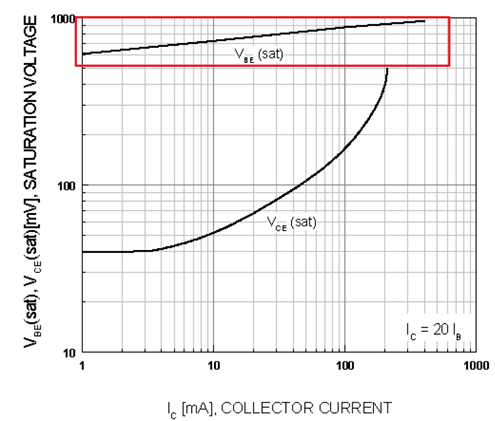Q1与Q2的发射极是连在一起的。如果Q1基极加正电压，Q2基极保持0V，那么实际上Q1的V_BE将会大于Q2的V_BE。我们已经知道了，假设I_E1的增加量为ΔI，那么I_E2的减小量也是ΔI，那么由于I_E与V_BE有对应关系，所以如果V_BE1的增加量为ΔV_BE，那么I_E2的减小量也是ΔV_BE。实际上，电路输出的变化量，也等于ΔV_BE乘上电路的增益。共射极放大电路本身就是以发射极电位为基准进行放大的。通过分析ΔV_BE，也可以解释差分放大的原理。
如果差分放大电路的两个三极管本身的V_BE不一样，那么在输出端会产生两个V_BE间的电压差乘以增益之后的电压，这个电压是个误差，正常工作状态下不希望看到，所以两个三极管V_BE的值要完全一致。
基极-发射极电压V_BE也容易受到温度影响，三极管的常见的温度系数是-2.5mV/℃，（如果电路板工作的温度变化了100摄氏度，那么V_BE可能变化了250mV，这就是之前的设计中始终为发射极电压留有裕量的原因）因此两个三极管的温度特性也要完全一样。如果三极管的温度特性完全一样，两个三极管的V_BE的温度变化会相互抵消，不会在输出中出现。有效的减小晶体管随温度变化而引起的零点漂移（温度漂移），是差分放大电路的优点之一。

### 直流电位分析与电阻取值

取Q1与Q2集电极的电流为0.1mA，则Q3集电极的电流为0.2mA。设定V_R6=2V，V_R1=V_R2=2.2V即可算出电路中所有的电阻的取值，与直流电位的情况。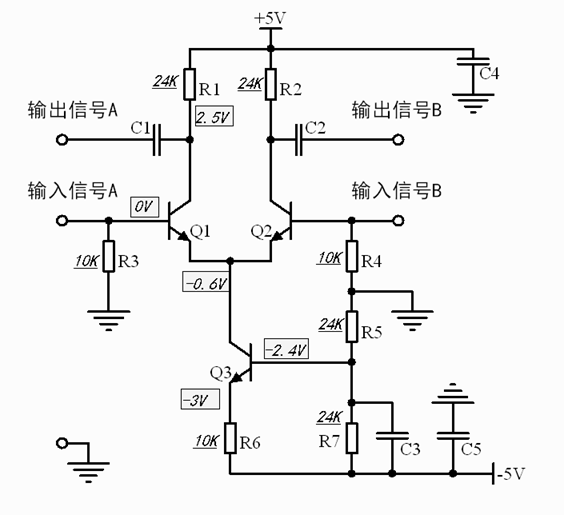根据共射极放大电路的知识，不难猜出其中R1与R2是输出阻抗，R3与R4是输入阻抗。

### 直流电位分析与电阻取值

以下是输入1KHz，100mV的正弦波，使用双通道示波器观察两路输出，并且用函数功能，观察“输出信号A-输出信号B”的波形。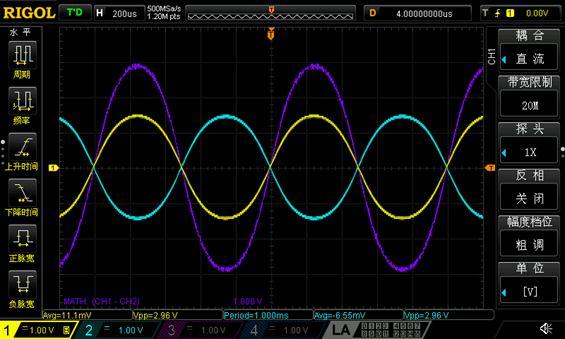展开全文• 结构与工作原理 工作组态与性质 放大电路 放大电路基础知识 基本共射放大电路的工作原理与分析方法 图解法分析: 等效电路法 静态工作点稳定问题 稳定原理 静态工作点计算 交流指标的计算 晶体单管放大电路三种组态 ...

### 半导体基本特性.

• 典型半导体有硅和锗以及砷化镓等.N型半导体为掺入五价杂质元素(如磷)的半导体, 主要载流子为电子(多数载流子).P型半导体为掺入三价杂质元素(如硼)的半导体,主要载流子为空穴(多数载流子).
• 对于P型半导体和N型半导体集合面, 例子薄层形成的空间电荷区称为PN结. 在空间电荷区,由于缺少多子, 所以也称耗尽层.
• 接触电位差(电位壁垒或势垒):
V ϕ = k T q l n ( N A N D n i 2 ) = V T l n ( N A N D n i 2 ) V_\phi=\frac{kT}{q}ln(\frac{N_AN_D}{n_i^2})=V_Tln(\frac{N_AN_D}{n_i^2}) ,
室温下(T=300K),Ge:0.2~0.3V
Si:0.6~0.7V
• 势垒宽度和掺杂浓度成反比.
• 当外加电压使PN结的P区电位高于N区电位, 称为正向电压,简称正偏,反之称为反向电压,称为反偏.

### 双极型晶体管

#### 结构与工作原理

• 由两个背靠背的PN节构成, 两种载流子参与导电----双极型结型晶体管.
• 两种类型: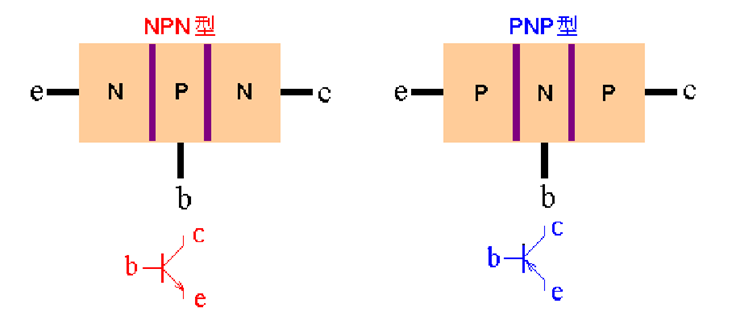• 结构特点: 发射区(e区)掺杂浓度最高; 集电区掺杂浓度低于发射区, 且面积大; 基区很薄, 一般在几微米到几十微米, 且掺杂浓度最低.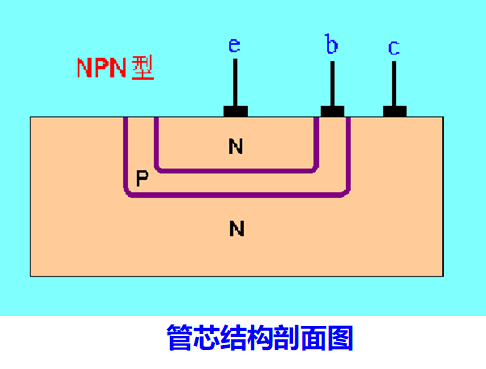• 四种工作状态:
• 放大: J E J_E 正偏, J C J_C 反偏
• 饱和: J E , J C J_E, J_C 正偏
• 截至: J E , J C J_E, J_C 反偏
• 反向: J E J_E 反偏, J C J_C 正偏
• NPN型晶体管中载流子传输示意: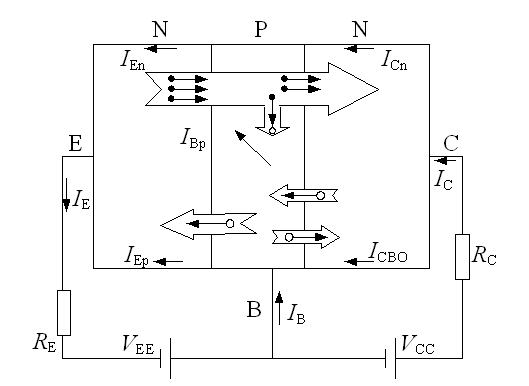1. 发射结电流: 发射结正偏, 电流方向为基区向发射区, 发射区中的多子(电子)向基区扩散, 形成电子扩散电流 I E n I_{En} ; 基区的多子:空穴同时也向发射区扩散, 形成空穴电流 I E p I_{Ep} , 二者的和就是发射极电流(发射区和基区的电流). 由于发射区掺杂浓度远高于基区掺杂浓度, 所以 I E n > > I E p I_{En}>>I_{Ep} .
I E = I E n + I E p ≈ I E n I_E=I_{En}+I_{Ep}\approx I_{En}
2. 基区内的电流: 由发射区扩散来的电子会在基区靠近发射区的边界处累积, 在基区形成浓度梯度, 该梯度将电子向集电区方向推动. 电子一边向集电区扩散(电流 I C n I_{Cn} ), 一边与基区内的多子(空穴)复合(电流 I B p I_{Bp} ). 而由于电源 V E E V_{EE} 的存在, 基区被不断补充多子(空穴),使基区空穴浓度保持不变.
I E n = I B p + I C n I_{En}=I_{Bp}+I_{Cn}
3. 集电区电流: 由扩散到边缘的电子漂移到集电区形成了电流 I C n I_{Cn} ; 另外, 由于集电结反偏, 基区和集电区的少子漂移形成了反向漂移电流 I C B O I_{CBO} , 其数值很小. I C = I C n + I C B O ≈ I C n I_{C}=I_{Cn}+I_{CBO}\approx I_{Cn}
又上图看出基极电流为 I B = I B p + I E p − I C B O ≈ I B p I_B=I_{Bp}+I_{Ep}-I_{CBO}\approx I_{Bp}
将晶体管看成一个节点, 其三个电极的电流满足节点方程 I E = I B + I C I_E=I_B+I_C

#### 工作组态与性质

• 晶体管三种连接方式的电流传输关系.
1. 共基极(CB, 共基)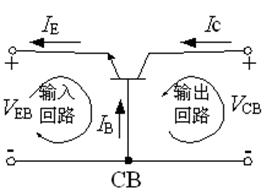输入电流为 I E I_E , 输出电流为 I C I_C , 引入参数 α ‾ , α ‾ \overline\alpha,\overlineα 定义为 α ‾ = I C n I E \overline\alpha=\frac{I_{Cn}}{I_E} ,得到 I C = α ‾ I E + I C B O I_C=\overline αI_E+I_{CBO} α称为共基直流放大系数, 范围典型值为0.95~0.995.为了使α趋近于1, 要求 I E p < < I E n , I B p < < I C n I_{Ep}<<I_{En},I_{Bp}<<I_{Cn} ,若忽略 I C B O I_{CBO} ,电流传输方程可简化为 I C ≈ α ‾ I E I_C\approx \overline \alpha I_E
2. 共发射极(CE, 共射)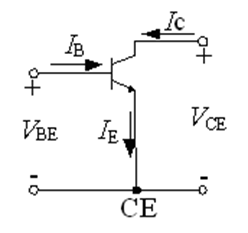输入电流为 I B I_B , 输出电流为 I C I_C , 根据上面推出的 I C I_C I E I_E 的关系, 其满足关系 I C = α ‾ ( I B + I C ) + I C B O I_C=\overline\alpha(I_B+I_C)+I_{CBO} 引入参数 β ‾ = α ‾ 1 − α ‾ , I C E O = 1 1 − α ‾ I C B O = ( 1 + β ‾ ) I C B O \overline\beta=\frac{\overline\alpha}{1-\overline\alpha}, I_{CEO}=\frac{1}{1-\overline\alpha}I_{CBO}=(1+\overline\beta)I_{CBO} ,
I C = β ‾ I B + I C E O I_C=\overline\beta I_B+I_{CEO}
I C E O I_{CEO} 称为穿透电流, 是基极开路( I B = 0 I_B=0 )时流过集电极与发射极的电流. 通常 I C E O I_{CEO} 很小, 上式化为 I C ≈ β ‾ I B I_C\approx \overline\beta I_B
β ‾ \overline\beta 称为共射直流放大系数, 通常一般为几十至几百.
3. 共集电极(CC,共集)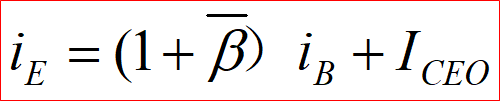• 晶体管静态特性曲线:
• 共射组态输入特性曲线: 该曲线描绘了当输出电压 v C E v_{CE} 固定时输入端电压( v B E v_{BE} )与电流( i B i_B )的关系.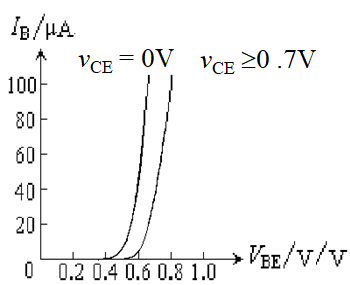• 当发射结加正向电压,( v B E > 0 v_{BE}>0 ),随着 v C E v_{CE} 的增大, 曲线右移.
• v C E = 0 v_{CE}=0 时,集电极与发射极短路,即发射结与集电结并联, 所以伏安特性曲线与PN结伏安特性曲线类似, 呈指数关系.
• v C E v_{CE} 增大时, 集电结由正偏逐渐变成反偏, 吸引电子能力加强, 从发射区注入到基区的电子更多的被集电结收集, 流向基极的电流 i B i_B 逐渐减小, 因此向右移动.
• v C E > 1 v_{CE}>1 时, 集电结的反向电压已经能将发射结注入到基区的电子大部分都收到集电区, 所以再增加 v C E v_{CE} , i B i_B 将不再明显减小而是略有减小, 使伏安特性曲线略向右移, 这是由于基区调宽效应引起的.
• 当发射结加反向电压( v C E < 0 v_{CE}<0 )时, 基极反向饱和电流很少, 当 v B E v_{BE} 向负值方向增大到 V ( B R ) E B O V_{(BR)EBO} 时, 发射结被击穿. V ( B R ) E B O V_{(BR)EBO} 称为发射结反向击穿电压, 由于发射区掺杂浓度很高, 因此属于齐纳击穿, 其值在-6V左右.
• 基区调宽效应: 通常将由 v C E v_{CE} 变化引起的基区有效宽度变化而导致电流变化的现象称为基区调宽效应.再工程上, 一般可以忽略基区调宽效应对输入特性的影响, 认为当 v C E v_{CE} 大于1以后输入曲线近似重合为一条.
• 输出特性曲线: 当输入电流 i B i_B 为某一常数时, 输出电流( i C i_C )和输出电压( v C E v_{CE} )的关系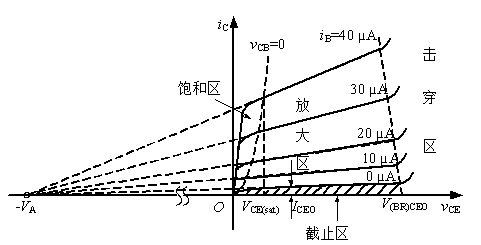根据外加电压不同, 图像分为4个区域, 饱和区, 放大区, 截止区和击穿区上图中 v C B = 0 v_{CB}=0 的虚线是 v C E = v B E v_{CE}=v_{BE} 各点的连线, 是放大区与饱和区的分界线. 因此可以通过该分界线获取 v B E v_{BE} 的值.
• 饱和区:　晶体管发射结与集电结都正偏的工作状态. 当 v C E v_{CE} 很小时, 集电极收集能力很弱, i C i_C 很小, v C E v_{CE} 稍有增加, 集电能力增强, 将更多基区电子拉到集电区, i C i_C 增长很快, 使 i C i_C v C E v_{CE} 影响很大, 所以此时曲线十分陡, 但随着 v C E v_{CE} 的增大, i C i_C 增速开始减缓.在饱和区, 集电极与发射极之间的电压降称为饱和电压, 用 V C E ( s a t ) V_{CE(sat)} 表示, 其大小与集电区体电阻和集电极电流有关, 对于小功率晶体管 V C E ( s a t ) V_{CE(sat)} 很小,其值常取0.3, 工程上近似于0, 即将集电极与发射极近似为短路.
• 放大区: 集电结反偏, 发射结正偏, 曲线基本水平稍有上翘. 当 v C E v_{CE} 大于1V后, 集电结的电场已足够强, 使发射区扩散到基区的电子绝大部分都到达了集电区, 因此再增加 v C E v_{CE} 的大小, i C i_C 几乎不变; 同时由于基区宽度调制效应, 当 v C E v_{CE} 增大时, 基区有效宽度减小, 这样基区内载流子复合机会减小, 使电流放大系数 β \beta 增大, 在 i B i_B 不变的情况下, i C i_C 增大. 但基区调宽效应对电流 i C i_C 的影响十分小, 故 i C i_C 的增大很小. 放大区输出特性曲线的上翘程度通常用晶体管输出电阻 r c e r_{ce} 表示, 定义为工作点Q处的曲线斜率倒数, 显然 r c e = V A + V C E Q I C Q ≈ V A I C Q r_{ce}=\frac{V_A+V_{CEQ}}{I_{CQ}}\approx\frac{V_A}{I_{CQ}}
• 厄尔利电压: 如果将输出特性曲线的每一条曲线向负轴方向延伸, 他们将近似在电压轴上交于一点A, 对应的电压 V A V_A , 称为厄尔利电压. V A V_A 与基区宽度 W B W_B 有关, W B W_B 越小, 基区调宽效应对 i C i_C 的影响越大, 曲线后半部分斜率越大, − V A -V_A 越靠近坐标轴, V A V_A 越小. 典型的NPN型小功率管的 V A V_A 为50~100V.
• 截止区: 集电结, 发射结反偏, 对应输出曲线 i B = 0 i_B=0 以下的区域. 此时集电结近似开路, 集电极电流即为穿透电流 I C E O I_{CEO} .
• 击穿区: 当集电结电压 v C B v_CB 增大到到一定值时, 集电结发生反向击穿, 造成集电极电流 i C i_C 剧增. 基区与集电区掺杂浓度低, 产生的反向击穿主要是雪崩击穿, 击穿电压较大. 由图可见击穿电压随着i_B的增大而减小. 因为 i B i_B 增大时, i C i_C 也增大, 通过集电极的载流子数目增多, 碰撞机会增大, 因而产生雪崩击穿所需的电压减小. i B = 0 i_B=0 时击穿电压用 V ( B R ) C E O V_{(BR)CEO} 表示, 是基极开路时集电极与发射极之间的击穿电压.

### 放大电路

#### 放大电路基础知识

• 放大电路的组成: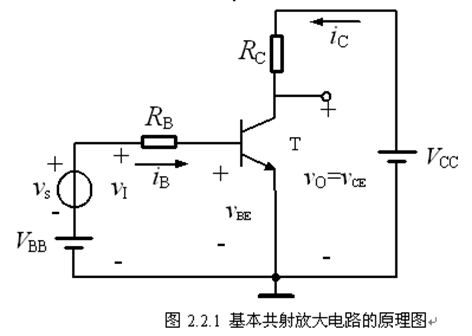(1). 直流工作点的设置:为了实现信号放大, 晶体管必须在信号的整个周期内都工作在放大区，　为此在输入端加一个合适的直流电压 V B B V_{BB} , 将交流小信号叠加在 V B B V_{BB} 上, 使作用于电路输入端的电压 v I = V B B + v s v_I=V_{BB}+v_s 始终大于发射结的阈值电压 V t h V_{th} .同时集电极电源电压 V C C V_{CC} 也要足够高, 保证集电极电流最大时的 v o = v C E = V C C − R C i C > v B E v_o=v_{CE}=V_{CC}-R_Ci_C>v_{BE}
(2). 交流信号的放大:设置Ｑ点之后, 幅度很小的交流电压 v s v_s 叠加在 V B B V_{BB} 上, 使电路的输入电压 v I = V B B + v s v_I=V_{BB}+v_s , 晶体管发射结对交流小信号可以等效为一个线性电阻 r b e r_{be} , 该电阻与 R B R_B 串联, 故发射结上的分得的交流电压为 v b e = r b e R B + r b e v_{be}=\frac{r_{be}}{R_B+r_{be}} , 于是 v B E = V B E Q + V b e m sin ⁡ ω t v_{BE}=V_{BEQ}+V_{bem}\sin \omega t 作用下的集电极电流 i C = I C Q + i c = I C Q + I c m sin ⁡ ω t i_C=I_{CQ}+i_c=I_{CQ}+I_{cm}\sin \omega t , 则输出电压为 v O = v C E = V C C − R C i C = ( V C C − R C I C Q ) − R C I c m sin ⁡ ω t = V C E Q + V o m sin ⁡ ( ω t + 18 0 o ) v_O=v_{CE}=V_{CC}-R_Ci_C=(V_{CC}-R_CI_{CQ})-R_CI_{cm}\sin \omega t=V_{CEQ}+V_{om}\sin(\omega t+180^o) ,可见, 只要 R C R_C 足够大, 就可以使输出信号 V o m V_{om} 比输入信号幅度 V s m V_{sm} 大得多, 从而实现了信号的放大.
• 放大电路的主要性能指标: 正弦稳态分析中, 电压, 电流用复数表示, R S R_S : 信号源内阻, R L R_L :负载电阻, 输入端电压电流: I ˙ i , V ˙ i \dot I_i,\dot V_i ,输出端电压电流: V ˙ o , I ˙ o \dot V_o,\dot I_o .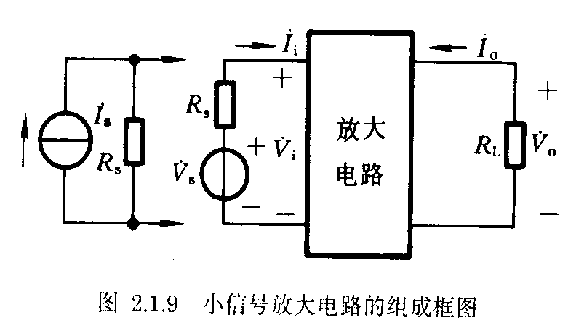• 输入阻抗: Z i = V ˙ i I ˙ i Z_i=\frac{\dot V_i}{\dot I_i} , 当在中频区(不考虑电抗), R i = V ˙ i I ˙ i R_i=\frac{\dot V_i}{\dot I_i} .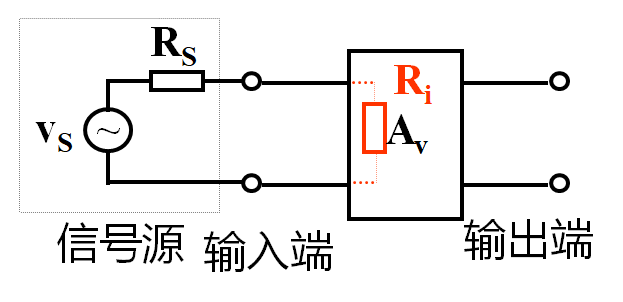• 输出阻抗: 当 R L R_L 开路, 输入信号源短路(保留 R S R_S 时)从输出端看入的电阻. Z o = V ˙ I ˙ ∣ R L = ∞ , V ˙ s = 0 Z_o=\frac{\dot V}{\dot I}|_{R_L=\infty,\dot V_s=0} 在输入端, 为了将信号尽可能多的送到输入端, 当输入量为电压时, 要求 R i > > R s R_i>>R_s , 尽量减小 R s R_s 分压, 称为恒压激励.当输入量为电流时, 要求 R i < < R s R_i<<R_s , 即所谓恒流激励.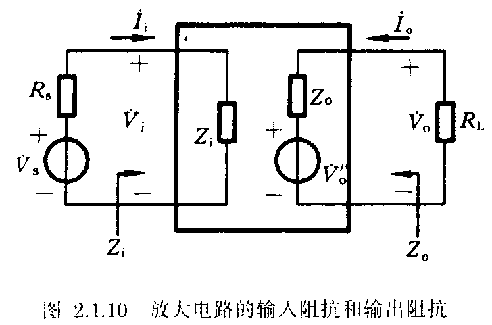• 计算 R o R_o 的方法:
1. 加压求流法: 将信号源短路, 但保留内阻, 保留受控源, 然后采用加压求流法计算出内阻.
2. 实验法:分别测出放大电路带负载 R L R_L 时的输出电压 V ˙ o \dot V_o 和空载( R L = ∞ R_L=\infty )时的电压 V ˙ o ′ \dot V_o' , R 0 = ( V ˙ o ′ V ˙ o − 1 ) ⋅ R L R_0=(\frac{\dot V_o'}{\dot V_o}-1)\cdot R_L
3. 开路电压除以短路电流: 分别测量开路电压和短路电流, 再相除.
• 增益(放大倍数), 定义为放大电路输出量与输入量的比值, 是直接衡量放大电路放大能力的指标. 根据输入量和输出量的不同, 可有四种放大电路, 即电压放大电路, 电流放大电路, 互阻放大电路和互导放大电路.
1. 电压增益: A ˙ v = V ˙ o V ˙ i \dot A_v=\frac{\dot V_o}{\dot V_i}
2. 源电压增益(考虑 R s R_s 的影响): A ˙ v s = R i R i + R s A ˙ v \dot A_{vs}=\frac{R_i}{R_i+R_s}\dot A_v
3. 电流增益: A ˙ i = I ˙ o I ˙ i \dot A_i=\frac{\dot I_o}{\dot I_i}
4. 源电流增益: A ˙ i = R s R s + R i A ˙ i \dot A_i=\frac{R_s}{R_s+R_i}\dot A_i
5. 功率增益: G P = P o P i = A v ⋅ A i G_P=\frac{P_o}{P_i}=A_v \cdot A_i

注: 放大倍数, 输入,输出电阻通常都是在正弦信号下的交流参数, 只有在放大电路处于放大状态时且输出不失真的条件下才有意义.

• 频率响应: 由于放大电路中电容电感的存在, 因此在不同频率下输出和输入间的增益, 相位不同 A ˙ v = A v ( ω ) e j φ ( ω ) \dot A_v=A_v(\omega)e^{j\varphi (\omega)}
• 最大输出功率: 非线性失真系数达到某数值时对应的最大输出电压和最大输出电流的乘积.
• 效率: 输出功率 P o P_o 与电源提供的平均功率 P D C P_{DC} 之比. η = P o P D C \eta=\frac{P_o}{P_{DC}}

#### 基本共射放大电路的工作原理与分析方法

• 基本共射放大电路工作原理: 设置Ｑ点之后, 幅度很小的交流电压 v s v_s 叠加在 V B B V_{BB} 上, 使电路的输入电压 v I = V B B + v s v_I=V_{BB}+v_s , 晶体管发射结对交流小信号可以等效为一个线性电阻 r b e r_{be} , 该电阻与 R B R_B 串联, 故发射结上的分得的交流电压为 v b e = r b e R B + r b e v_{be}=\frac{r_{be}}{R_B+r_{be}} , 于是 v B E = V B E Q + V b e m sin ⁡ ω t v_{BE}=V_{BEQ}+V_{bem}\sin \omega t 作用下的集电极电流 i C = I C Q + i c = I C Q + I c m sin ⁡ ω t i_C=I_{CQ}+i_c=I_{CQ}+I_{cm}\sin \omega t , 则输出电压为 v O = v C E = V C C − R C i C = ( V C C − R C I C Q ) − R C I c m sin ⁡ ω t = V C E Q + V o m sin ⁡ ( ω t + 18 0 o ) v_O=v_{CE}=V_{CC}-R_Ci_C=(V_{CC}-R_CI_{CQ})-R_CI_{cm}\sin \omega t=V_{CEQ}+V_{om}\sin(\omega t+180^o) ,可见, 只要 R C R_C 足够大, 就可以使输出信号 V o m V_{om} 比输入信号幅度 V s m V_{sm} 大得多, 从而实现了信号的放大. 见共射放大电路的工作波形, 可见输出电压与输入电压相位相反: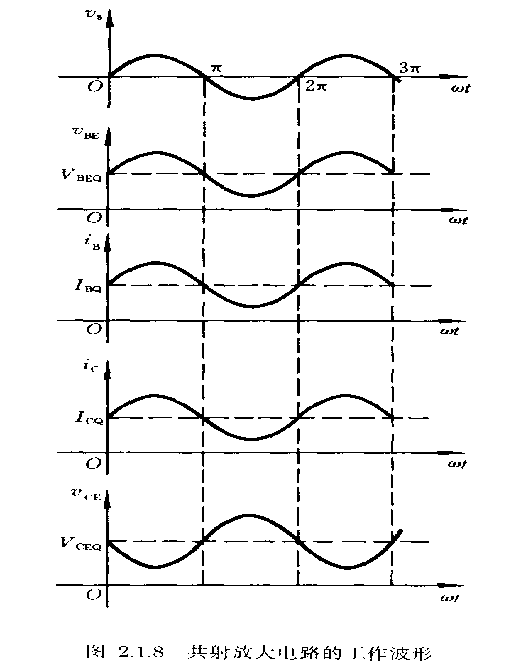##### 图解法分析:

一. 静态工作点分析
(1). 先画出直流通路图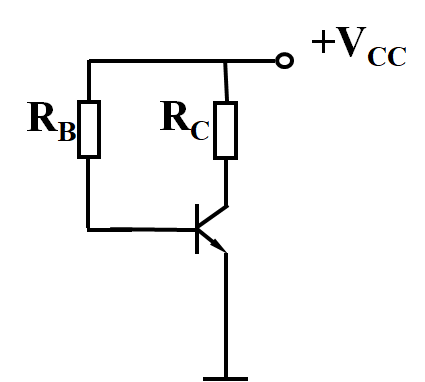(2). 在输入特性曲线上做出直流负载线, 画出 I B Q , V B E Q I_{BQ}, V_{BEQ} 的图像: I B = f 1 ( V B E ) I B = V C C − V B E R B I_B=f_1(V_{BE})\\[2ex] I_B=\frac{V_{CC}-V_{BE}}{R_B}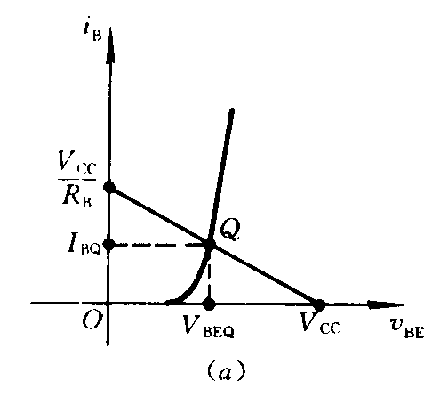直流负载线与输入特性曲线交点即为静态工作点Q, 从而可以确定 I B Q , V B E Q I_{BQ},V_{BEQ} 的值, 工程上经常估算 I B Q I_{BQ} 的值, I B Q = V C C − V B E Q R B I_{BQ}=\frac{V_{CC}-V_{BEQ}}{R_B}
对小功率锗管区 V B E Q = 0.2 ～ 0.3 V V_{BEQ}=0.2 ～0.3V , 小功率硅管 0.6 ～ 0.7 V 0.6～0.7V .
(3). 在输出特性曲线上做出回路的直流负载线, 求出 I C Q , V C E Q I_{CQ},V_{CEQ} . 在输出回路中, 静态工作点既满足晶体管的输出特性曲线, 又满足外回路方程 V C E = V C C − I C R C V_{CE}=V_{CC}-I_CR_C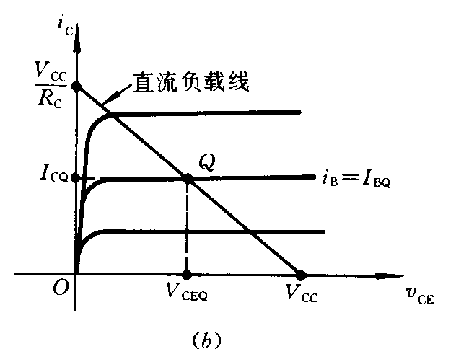画出对应的直线, 在找到 I B = I B Q I_B=I_{BQ} 的输出曲线, 这两条线的交点即为静态工作点, 从而可以确定出 I C Q , V C E Q I_{CQ},V_{CEQ} , 这样Q点就确定了.
二. 动态图解分析
(1). 画出放大电路的交流通路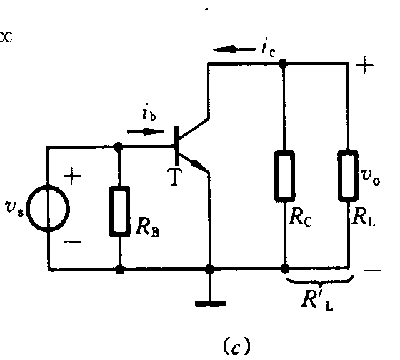(2). 根据输入信号求出 v B E , i B v_{BE},i_B 的波形. 将 v B E = V B E Q + v s v_{BE}=V_{BEQ}+v_s 画在输入特性曲线的下方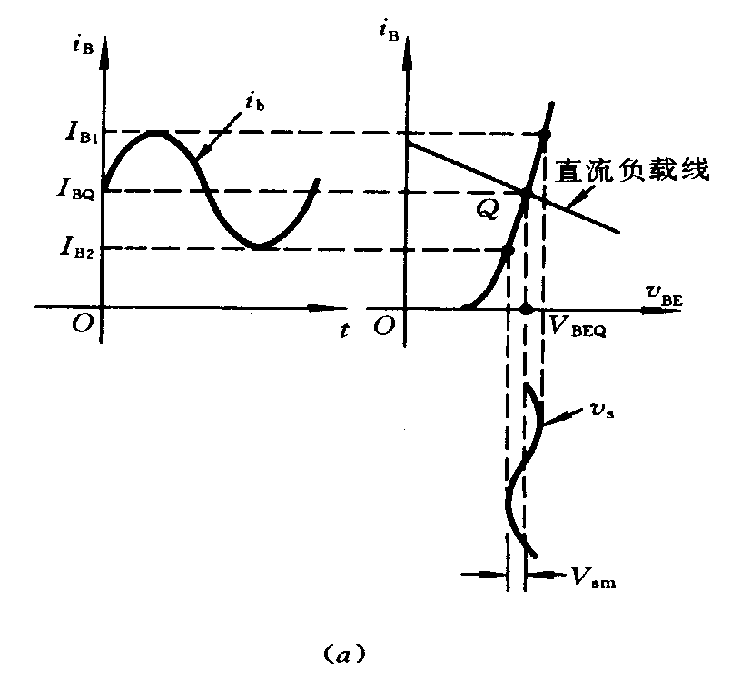根据 v B E v_{BE} 的变化规律, 便可从输入特性画出对应的 i B , i b i_B,i_b 的波形. i B i_B 的最大值为 I B 1 I_{B1} , 最小值为 I B 2 I_{B2} ,他们决定了输出特性曲线的工作范围.
(3). 在输出特性曲线上做交流负载线, 求 i C 及 v C E i_C及v_{CE} 的波形. 设晶体管集电极的交流等效电阻为 R L ′ R_L' ,则 R L ′ = R C / / R L R_L'=R_C//R_L ,由交流通路图可写出输出回路方程式 v c e = i c R L ′ ( 交 流 方 程 ) v C E = v c e + V C E Q i C = i c + I C Q v_{ce}=i_{c}R_L'(交流方程)\\[2ex] v_{CE}=v_{ce}+V_{CEQ}\\[2ex]i_{C}=i_{c}+I_{CQ} i C = − 1 R L ′ v C E + V C E Q + I C Q R L ′ R L ′ ( 全 值 方 程 ) i_C=-\frac{1}{R_L'}v_{CE}+\frac{V_{CEQ}+I_{CQ}R_L'}{R_L'}(全值方程)
将上式确定的曲线画到输出特性曲线上,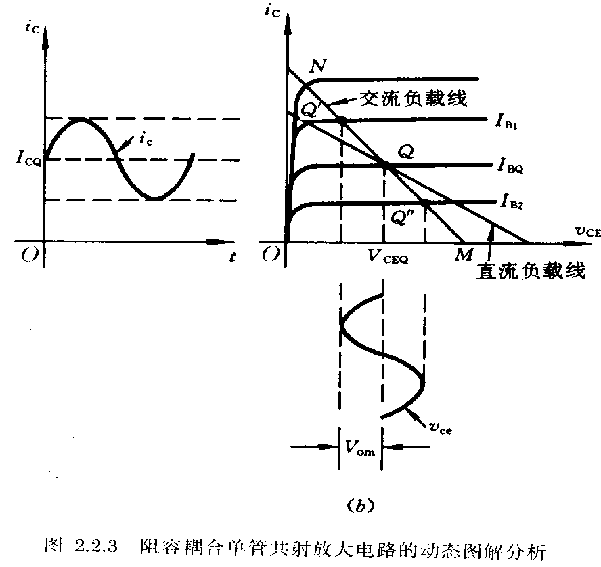(4). 求 i C , v C E i_C,v_{CE} 波形: 基极电流 i B i_B 在时刻变化, 每一个 i B i_B 对应一条输出特性曲线, 该曲线与交流负载线的交点便是此 i B i_B 下的工作点. 当 i B i_B 分别取 I B 1 , I B 2 I_{B1},I_{B2} 时, 两条输出特性曲线和负载分别交于 Q ′ , Q ′ ′ Q',Q'' , 晶体管的工作范围处于 Q ′ , Q ′ ′ Q',Q'' 之间. 由此可画出 i C , v C E i_C,v_{CE} 的波形.
此时, 从图中可以看出 v s , i b , v b e , i c v_s,i_b,v_{be},i_c 同相位, v o = v c e v_o=v_{ce} v s v_s 反向. 由图中 V s m , V o m V_{sm},V_{om} 便可求出 A v s = V o m V s m A_{vs}=\frac{V_{om}}{V_{sm}}
三. 静态工作点的选择与波形失真及动态范围

• 由上图可知静态工作点的选取应满足下列条件 { I C Q > I c m + I C E O V C E Q > V c m + V C E ( s a t ) \begin{cases} I_{CQ}>I_{cm}+I_{CEO}\\[2ex] V_{CEQ}>V_{cm}+V_{CE(sat)} \end{cases}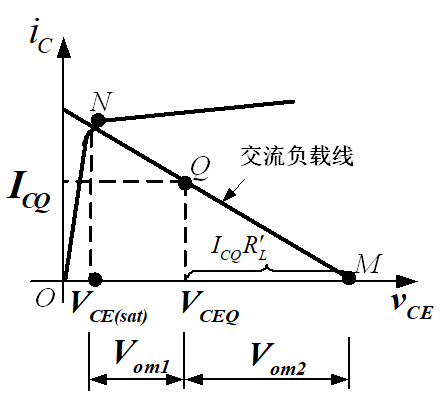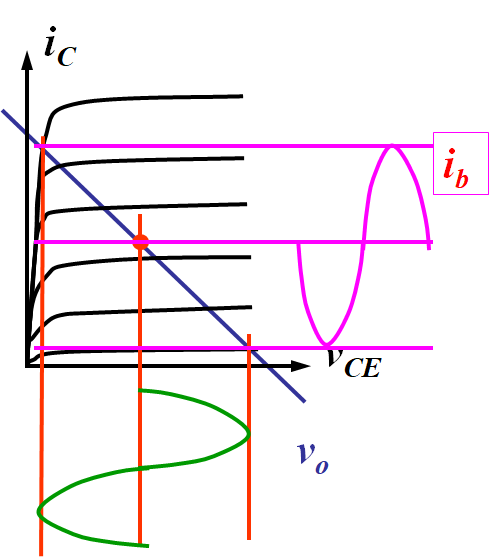由上图可知, 若Q点过高( I C Q I_{CQ} 偏大), 放大电路容易进入饱和区,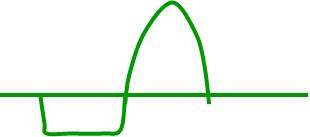(饱和失真)
Q点过低, 放大电路易进入截止区,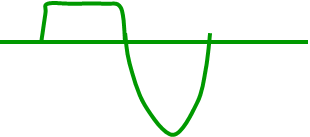(截止失真)
因此一旦 V C C , R C , R L V_{CC},R_C,R_L 确定, Q点应该选择交流负载线NM的中点. 这样放大电路的动态范围最大.
##### 等效电路法

一. 静态工作点估算.
1. 晶体管的简化直流模型.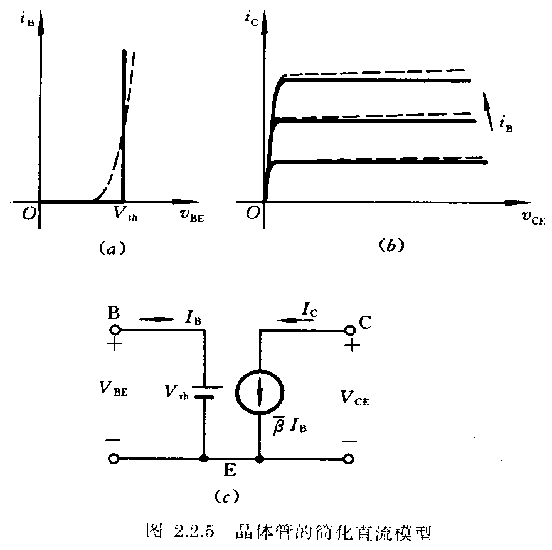输入回路用恒压源 V t h V_{th} 等效, 输出端用一受控源表示 I C = β I B I_C=\beta I_B .由上图所示的输入, 输出回路易得
{ I B Q = V C C − V B E Q R B I C Q = β I B Q V C E Q = V C C − R C I C Q \begin{cases} I_{BQ}=\frac{V_{CC}-V_{BEQ}}{R_B}\\[2ex] I_{CQ}=\beta I_{BQ}\\[2ex] V_{CEQ}=V_{CC}-R_CI_{CQ}\\[2ex] \end{cases}

• 判断是否处于饱和区: 由于在放大区和饱和区的临界点处 I C = β I B I_C=\beta I_B 仍然成立, 将临界点处的电流用 I B S , I C S I_{BS},I_{CS} 表示, 则有 I B S = I C S / β = ( V C C − V C E ( s a t ) ) / ( β R C ) I_{BS}=I_{CS}/\beta=(V_{CC}-V_{CE_(sat)})/(\beta R_C) 只要 I B > I B S I_B>I_{BS} , 该电路就工作在饱和区.

eg: P65.2.3.1
二. 晶体管混合 π \pi 模型及交流指标的运算.

1. 混合 π \pi 模型的导出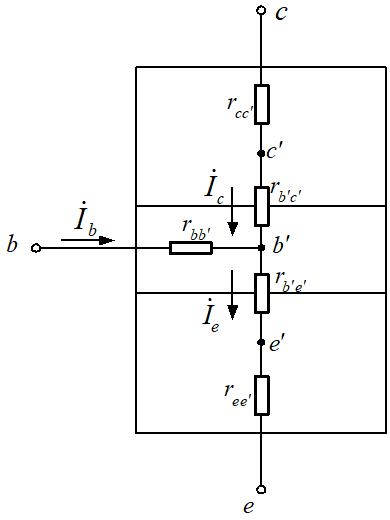晶体管结构示意图
上图中分别用 b ′ , e ′ , c ′ b',e',c' 表示晶体管三个区内部的等效节点, r b b ′ , r c c ′ r e e ′ r_{bb'},r_{cc'}r_{ee'} 分别表示三个区的体电阻; 对于交流小信号, 发射结用其Q点的动态电阻 r b ′ e ′ r_{b'e'} 表示, 集电结用其动态电阻 r b ′ c ′ r_{b'c'} 表示. 由于发射结掺杂浓度高, 集电区的结面积大, r e e ′ , r c c ′ r_{ee'},r_{cc'} 较小, 基区薄且掺杂浓度低, r b b ′ r_{bb'} 较大, 故在混合 π \pi 模型中, 只保留了基区体电阻 r b b ′ r_{bb'} ,发射结和集电结的动态电阻 r b ′ e ′ , r b ′ c ′ r_{b'e'},r_{b'c'} , 可表示为 r b ′ e , r b ′ c r_{b'e},r_{b'c} 易知 r b ′ e = V T I B Q = ( 1 + β ) V T I E Q r_{b'e}=\frac{V_T}{I_{BQ}}=(1+\beta)\frac{V_T}{I_{EQ}}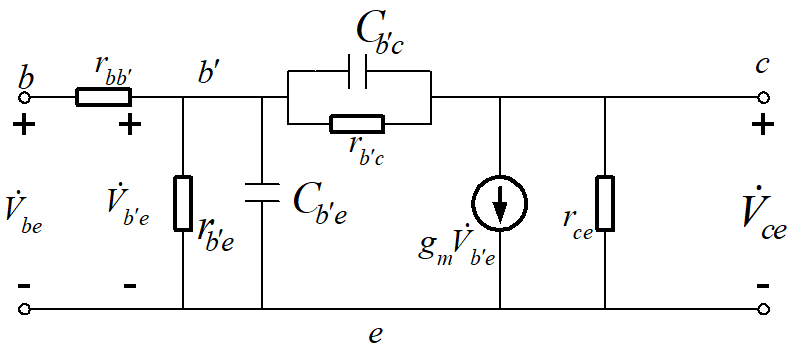晶体管混合 π \pi 模型中, 使用电容 C b ′ c , C b ′ e C_{b'c},C_{b'e} 等效集电结, 发射结的电容. 再放大区 , i C i_C i B i_{B} 控制, 而 i B i_B 是受发射结电压 V ˙ b ′ e \dot V_{b'e} 控制的, 因此在模型中用一个受 V ˙ b ′ e \dot V_{b'e} 控制的电流源 g m V ˙ b ′ e g_m\dot V_{b'e} 表示晶体管的输出, g m g_m 称为跨导, 表示输入电压对输出电流的控制能力; r c e r_{ce} 是描述基区调宽效应的输出电阻. 低频区和中频区中, 由于 r b ′ c r_{b'c} 反偏很大, 因此当作开路, 同时忽略电容的作用, 因此低中频的混合 π \pi 模型如下图所示: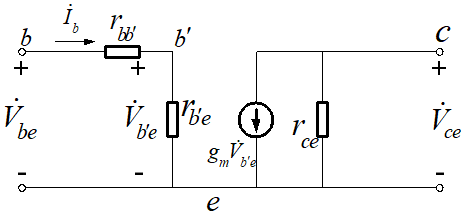上图中 r b b ′ r_{bb'} r b ′ e r_{b'e} 串联, 总电阻用 r b e r_{be} 表示, 即 r b e = r b b ′ + r b ′ e = r b b ′ + ( 1 + β ) V T I E Q r_{be}=r_{bb'}+r_{b'e}=r_{bb'}+(1+\beta)\frac{V_T}{I_{EQ}}
而在高频电路中, C b ′ e C_{b'e} 主要是扩散电容, 数值较大, C b ′ c C_{b'c} 主要是势垒电容, 数值较小, r b ′ e > > 1 / ( ω C b ′ e ) r_{b'e}>>1/(\omega C_{b'e}) ,忽略 r b ′ c r_{b'c} , 故高频混合 π \pi 模型如下图: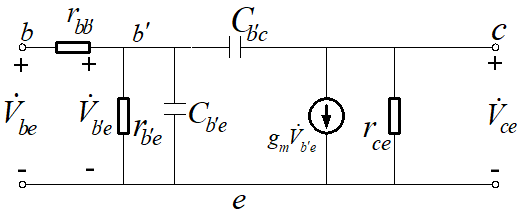2. 混合 π \pi 模型参数的计算: 一般情况下, r b b ′ r_{bb'} 器件手册会给出, r b ′ e r_{b'e} 可由 r b e = r b b ′ + r b ′ e = r b b ′ + ( 1 + β ) V T I E Q r_{be}=r_{bb'}+r_{b'e}=r_{bb'}+(1+\beta)\frac{V_T}{I_{EQ}} 计算出, r c e r_{ce} 可由 r c e = V A + V C E Q I C Q ≈ V A I C Q r_{ce}=\frac{V_A+V_{CEQ}}{I_{CQ}}\approx\frac{V_A}{I_{CQ}} ( V A V_A 为厄尔利电压), 但其一般较大, 经常忽略. 可以证明 g m r b ′ e = β g_mr_{b'e}=\beta , 联立有 g m = β ( 1 + β ) V T I E Q = α I E Q V T = I C Q V T g_m=\frac{\beta}{(1+\beta)\frac{V_T}{I_{EQ}}}=\frac{\alpha I_{EQ}}{V_T}=\frac{I_{CQ}}{V_T} 对于低中频的混合 π \pi 模型, 可知 g m V ˙ b ′ e = g m r b ′ e I ˙ b = β I ˙ b g_m\dot V_{b'e}=g_mr_{b'e}\dot I_b=\beta \dot I_b , 所以输出回路的受控源也通常用 β I ˙ b \beta \dot I_b 表示. 各参数在频率低于 f T / 3 f_T/3 时基本与频率无关, 因此他的频率适用范围是 f < f T / 3 f<f_T/3 .
3. 使用混合 π \pi 模型计算放大电路的动态性能指标
一般步骤:
(1). 确定静态工作点
(2). 求出放大电路在Q点的混合 π \pi 模型参数 β , r b ′ e \beta, r_{b'e}
(3). 画出交流通路图, 用混合 π \pi 模型代替三极管, 得到小信号交流等效电路.
(4). 求解放大电路性能指标.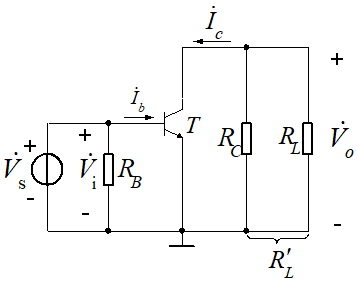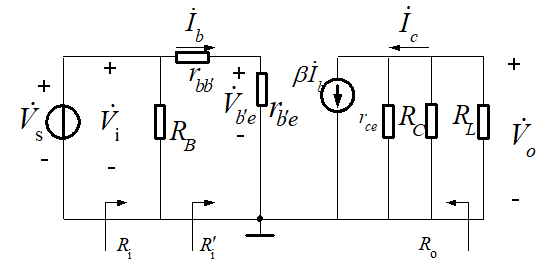• 电压增益: 总负载电阻 R L ′ = r c e / / R C / / R L R_L'=r_{ce}//R_C//R_L , 不难得出 V ˙ o = − β I ˙ b R L ′ A ˙ v = V ˙ o V i = − β R L ′ r b e \dot V_o=-\beta \dot I_bR_L'\\[2ex]\dot A_v=\frac{\dot V_o}{V_i}=-\frac{\beta R_L'}{r_{be}}
• 输入电阻: 按定义可得 R i ′ = V ˙ i I ˙ b = r b e R i = V ˙ i I ˙ i = R B / / r b e R_i'=\frac{\dot V_i}{\dot I_b}=r_{be}\\[2ex]R_i=\frac{\dot V_i}{\dot I_i}=R_B//r_{be}
• 输出电阻: R o = V ˙ I ˙ = r c e / / R C R_o=\frac{\dot V}{\dot I}=r_{ce}//R_C
eg: P69.2.3.2

#### 静态工作点稳定问题

##### 稳定原理
• 温度对静态工作点的影响: 温度升高时, 晶体管 I C B O , β I_{CBO}, \beta 增大, v B E v_{BE} 减小, 其结果为静态电流 I C Q I_{CQ} 增大.
• 典型的Q点稳定电路如下(分压式电流负反馈Q点稳定电路):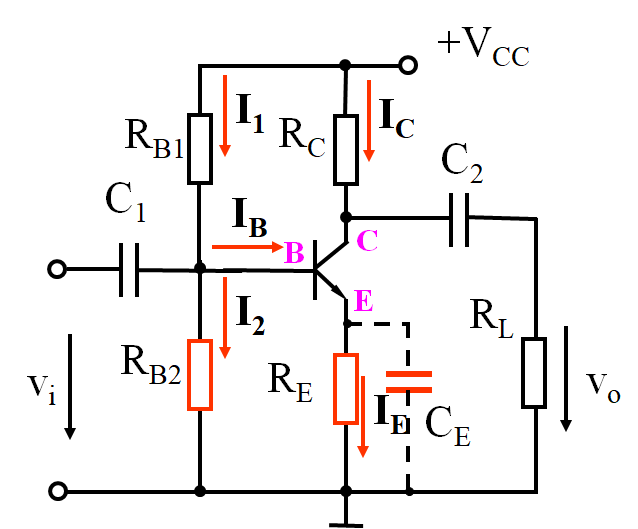电路结构上采取了两个措施, 一是采用分压式电路固定基极电位, 二是发射结接入电阻 R E R_E , 实现自动调节.具体原理是: 当温度升高时, I C Q ( I E Q ) I_{CQ}(I_{EQ}) 增加, 电阻 R E R_E 压降增加, 射极电位 V E Q V_{EQ} 增加, 而由于 V B Q V_BQ 的固定, 导致发射结上的压降 V B E Q V_{BEQ} 减小, 再根据二极管的RC曲线, 电流 I B Q I_{BQ} 也减小, 从而使 I C Q I_{CQ} 减小. 结果牵制了 I C Q I_{CQ} 的增加, 维持其基本不变. 这种调节作用称为反馈, 由于反馈的结果使电流输出量减小, 故称为电流负反馈, 又由于再直流电路中, 故称为直流负反馈, R E R_E 为负反馈电阻, R E R_E 越大, 负反馈越强, I C Q I_{CQ} 的稳定性越好, 但对于一定的集电极电流 I C I_C , 由于 V C C V_{CC} 的限制, R E R_E 太大会使晶体管进入饱和区, 电路将不能正常工作. 上述过程可简述如下: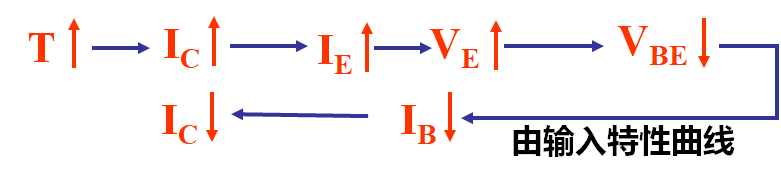##### 静态工作点计算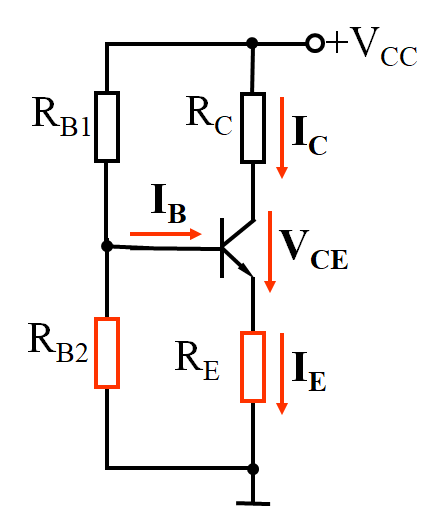• 近似估算: 已知 I B 1 > > I B , V B Q ≈ R B 2 R B 1 + R B 2 V C C I_{B1}>>I_B,V_{BQ}\approx \frac{R_{B2}}{R_{B1}+R_{B2}}V_{CC} ,可得 I C Q ≈ I E Q = V B Q − V B E Q R E I B Q = I E Q 1 + β = V B Q − V B E Q R E ( 1 + β ) V C E Q = V C C − I C Q ( R C + R E ) I_{CQ}\approx I_{EQ}=\frac{V_{BQ}-V_{BEQ}}{R_E}\\[2ex]I_{BQ}=\frac{I_{EQ}}{1+\beta}=\frac{V_{BQ}-V_{BEQ}}{R_E(1+\beta)}\\[2ex]V_{CEQ}=V_{CC}-I_{CQ}(R_C+R_E)
• 精确计算: 无论电路参数是否满足 I B 1 > > I B , R E I_{B1}>>I_B,R_E 的负反馈作用都存在, 将直流通路图中基极偏置电路 V C C , R B 1 , R B 2 V_{CC},R_{B1},R_{B2} 用戴维南定理等效成一个电压源, 其内阻 R B R_B ,电压 V B B V_{BB} 分别是 { V B B = R B 2 R B 1 + R B 2 V C C R B = R B 1 / / R B 2 \begin{cases}\\V_{BB}=\frac{R_{B2}}{R_{B1}+R_{B2}}V_{CC}\\[2ex]R_B=R_{B1}//R_{B2}\end{cases}
再将晶体管用简化直流模型替换, 得到下图电路, 输入回路的方程为:
V B B = I B Q R B + V B E Q + R E ( I B + β I B ) V_{BB}=I_{BQ}R_B+V_{BEQ}+R_E(I_B+\beta I_B) 则有
{ I B Q = V B B − V B E Q R B + ( 1 + β ) R E I C Q = β I B Q V C E Q ≈ V C C − ( R C + R E ) I C Q \begin{cases} I_{BQ}=\frac{V_{BB}-V_{BEQ}}{R_B+(1+\beta)R_E}\\[2ex] I_{CQ}=\beta I_{BQ}\\[2ex] V_{CEQ}\approx V_{CC}-(R_C+R_E)I_{CQ} \end{cases}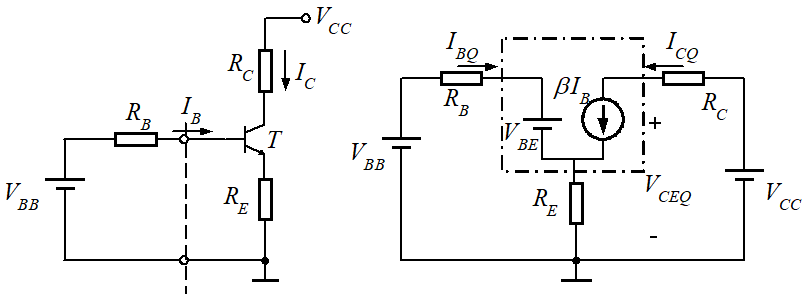• 两种方法对比: 可以看出当 ( 1 + β ) R E > > R B (1+\beta)R_E>>R_B 时, 两种计算方法的 I B Q , I C Q , V C E Q I_{BQ},I_{CQ},V_{CEQ} 相同, 因此可用 ( 1 + β ) R E (1+\beta)R_E R B 1 / / R B 2 R_{B1}//R_{B2} 的大小关系来判断式 I 1 > > I B I_1>>I_B 是否成立, 成立时则用近似计算.
##### 交流指标的计算

画出交流通路图及交流等效电路: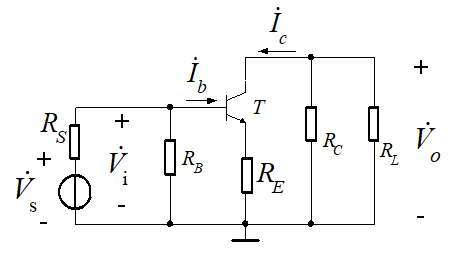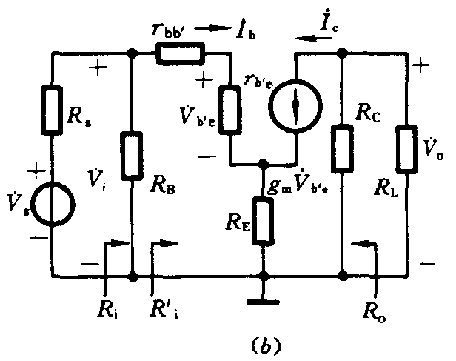其中 R L ′ = R C / / R L , R B = R B 1 / / R B 2 R'_L=R_C//R_L,R_B=R_{B1}//R_{B2} 由电路可列出
V ˙ o = − β I ˙ b R L ′ V ˙ i = I ˙ b ( r b b ′ + r b ′ e ) + ( I ˙ b + β I ˙ b ) R E = I ˙ b r b e + ( 1 + β ) I ˙ b R E A ˙ v = V ˙ o V ˙ i = β R L ′ r b e + ( 1 + β ) R E R i ′ = V ˙ i I ˙ b = r b e + ( 1 + β ) R E R i = R i ′ / / R B = R B / / [ r b e + ( 1 + β ) R E ] A ˙ v s = R i R i + R s A ˙ v \dot V_o=-\beta \dot I_b R_L'\\[2ex] \dot V_i=\dot I_b(r_{bb'}+r_{b'e})+(\dot I_b+\beta \dot I_b)R_E=\dot I_{b}r_{be}+(1+\beta)\dot I_bR_E\\[2ex] \dot A_v=\frac{\dot V_o}{\dot V_i}=\frac{\beta R_L'}{r_{be}+(1+\beta)R_E}\\[2ex] R_i'=\frac{\dot V_i}{\dot I_b}=r_{be}+(1+\beta)R_E\\[2ex] R_i=R_i'//R_B=R_B//[r_{be}+(1+\beta)R_E]\\[2ex] \dot A_{vs}=\frac{R_i}{R_i+R_s}\dot A_v
此外, 忽略 r c e r_{ce} 后, 受控电流源组织为无穷大, 因而输出电阻为 R o ≈ R C R_o\approx R_C
从上面的式子可以看出, R E R_E 的加入使 R i R_i 增大, A ˙ v \dot A_v 都减小, 因此可以在 R E R_E 两侧并联一个大电容 C E C_E , 接入 C E C_E 后, 对电路工作点没有影响, 交流通路中的 R E R_E 被旁路, 与阻容耦合放大电路的交流等效电路完全相同, 因此交流指标分别为 A ˙ v = − β R L ′ r b e R i = R B / / r b e R o ≈ R C \dot A_v=-\frac{\beta R_L'}{r_{be}}\\[2ex] R_i=R_B//r_{be}\\[2ex] R_o\approx R_C
eg: P73.2.4.1

#### 晶体单管放大电路三种组态

##### 共集放大电路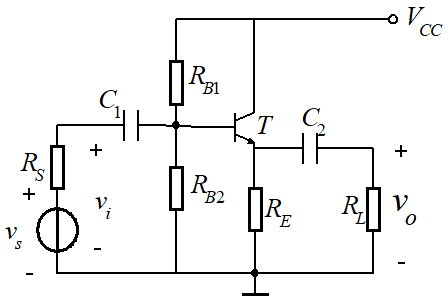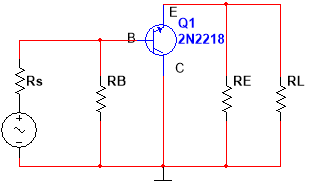一. 动态指标
R L ′ = R L + R E R_L'=R_L+R_E ,图中忽略 r c e r_{ce}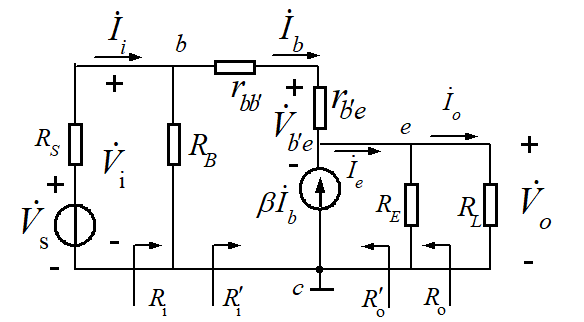• 电压增益与电流增益
V ˙ o = I ˙ e R L ′ = ( β + 1 ) I ˙ b R L ′ V ˙ i = r b e I ˙ b + V ˙ o A ˙ v = V ˙ o V ˙ i = ( 1 + β ) R L ′ r b e + ( 1 + β ) R L ′ \dot V_o=\dot I_eR_L'=(\beta+1)\dot I_bR_L'\\[2ex] \dot V_i=r_{be}\dot I_b+\dot V_o\\[2ex] \dot A_v=\frac{\dot V_o}{\dot V_i}=\frac{(1+\beta)R_L'}{r_{be}+(1+\beta)R_L'}
特点: 增益为同相. 电压增益近似为1(故称为电压跟随器, 射极跟随器), 电流增益较高, 故电路仍有功率放大作用.
• 输入电阻, 输出电阻
R i ′ = V ˙ i I ˙ b = r b e + ( β + 1 ) R L ′ R i = R i ′ / / R B = R B / / [ r b e + ( β + 1 ) R L ′ ] R s ′ = R s / / R B R o ′ = = V ˙ I ˙ ′ = − I ˙ b ( r b e + R s ′ ) − ( I ˙ b + β I ˙ b ) = R s ′ + r b e 1 + β R o = R o ′ / / R E = R E / / ( R s ′ + r b e 1 + β ) R_i'=\frac{\dot V_i}{\dot I_{b}}=r_{be}+(\beta+1)R_L'\\[2ex] R_i=R_i'//R_B=R_B//[r_{be}+(\beta+1)R_L']\\[2ex] R_s'=R_s//R_B\\[3ex] R_o'==\frac{\dot V}{\dot I'}=\frac{-\dot I_b(r_{be}+R_s')}{-(\dot I_b+\beta\dot I_b)}=\frac{R_s'+r_{be}}{1+\beta}\\[2ex] R_o=R_o'//R_E=R_E//(\frac{R_s'+r_{be}}{1+\beta})
特点: 输入电阻比共射放大电路高得多, 一般可达几十千欧到几百千欧. 输出电阻很小, 且与信号源内阻有关, 因此有很强的带负载能力.

二. 主要应用
共集放大电路输入电阻大, 输出电阻小, 电流驱动能力强, 因而从信号源索取的电流小而带负载能力强, 故常用于多级放大电路的输入级和输 出级; 也可用它来连接两电路, 减小电路直接相连造成的影响, 起缓冲的作用, 称为缓冲级或隔离级.
eg: P76.2.5.1

##### 共基放大电路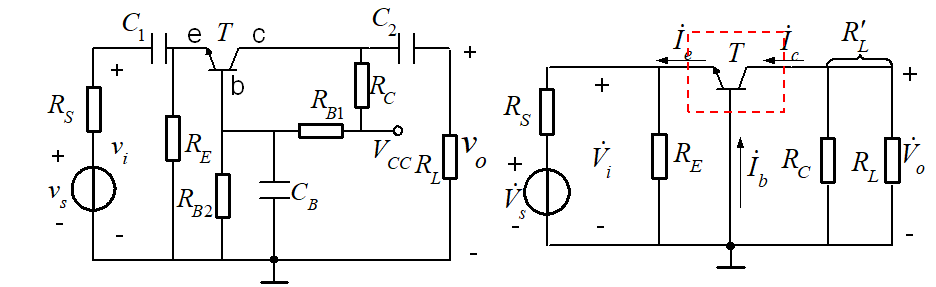R L ′ = R C / / R L R_L'=R_C//R_L , 忽略 r c e r_{ce}

• 电压增益与电流增益: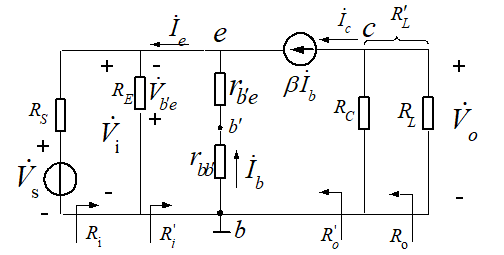V ˙ o = − β I ˙ b R L ′ V ˙ i = − I ˙ b ( r b b ′ + r b ′ e ) = − I ˙ b r b e A ˙ v = β R L ′ r b e \dot V_o=-\beta \dot I_bR_L'\\[2ex] \dot V_i=-\dot I_b(r_{bb'}+r_{b'e})=-\dot I_br_{be}\\[2ex] \dot A_v=\frac{\beta R_L'}{r_{be}}
特点: 输入电压与输出电压通相, 增益与共射放大电路相同, 输入电流为 i E i_E ,输出电流为 i C i_C , 故无电流放大能力, 但有足够的电压放大能力, 可以实现功率放大.

• 输入与输出电阻
R i ′ = V ˙ i I ˙ e = r b e 1 + β R i = R i ′ / / R E = R E / / r b e 1 + β R_i'=\frac{\dot V_i}{\dot I_e}=\frac{r_{be}}{1+\beta}\\[2ex] R_i=R_i'//R_E=R_E//\frac{r_{be}}{1+\beta}
若不考虑电阻 R C R_C 的作用, 则 R o = r c b R_o=r_{cb} , 晶体管的 r c b r_{cb} r c e r_{ce} 大得多, 如果考虑 R C R_C , 则 R o = r c b / / R C ≈ R C R_o=r_{cb}//R_C\approx R_C
故共基放大电路输出电阻与共射放大电路相同.
##### 三种组态比较
• 共射电路既能放大电流又能放大电压, 输入电阻在三种组态中居中,输出电阻大, 频带窄, 常作为低频电压放大电路的单元电路
• 共集电路只能放大电流不能放大电压, 是三种接法中输入电阻最大, 输出电阻最小的电路, 并具有电压跟随特点, 常用于多级放大电路的输入级, 输出极和缓冲极, 在功率放大电路也常采用射极输出形式
• 共集放大电路只能放大电压不能放大电流, 输出电阻小, 电压增益和输出电阻与共射电路相同, 频率特性是三种电路中最好的, 常用于宽频带放大电路.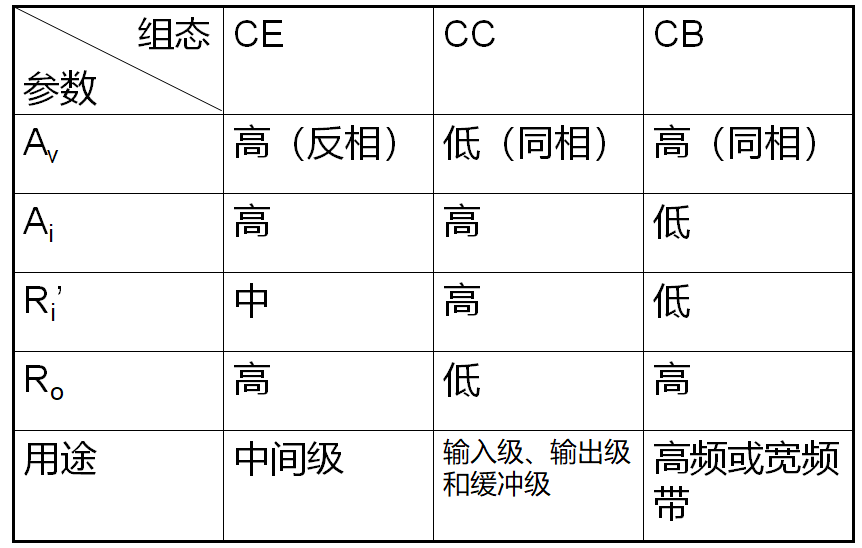### 差分放大电路

#### 差分(差动)放大器组成及特性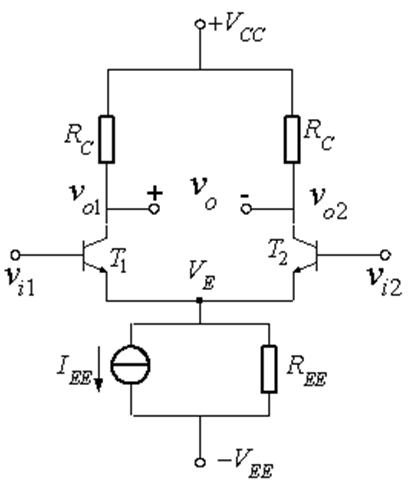• 组成: 电路左右对称, T 1 , T 2 T_1,T_2 特性一致, 故称 T 1 , T 2 T_1,T_2 为差分对管, I E E , R E E I_{EE},R_{EE}